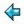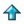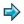### just.m

Text formatting program (DT), reformats text to a specified width, with line-fill

To try this out from within a Miranda session, say e.g.

```      just 60 (read "file")
```
where "file" contains some text you want to reformat.

You could also make it into a UNIX filter -- see the example `mrev'.

```%export just

||----------------------------------------------------------------------||
|| in this program we move between three different representations of   ||
|| text - as a flat list of characters, including spaces and newlines   ||
|| - as a list of lines (containing spaces but not newlines)            ||
|| - and as a list of list of words.                                    ||
||----------------------------------------------------------------------||

text == [char]
line == [char]
word == [char]

just::num->text->text  ||the main function
just n = concat.map(reformat n).paras.map words.lines

||lines::text->[line]
||lines is defined in <stdenv> - it breaks a string into lines,
||removing the newline characters

paras::[[word]]->[[word]]
||make each paragraph into one long line, by joining adjacent
||non-blank lines
paras (a:b:x) = paras ((a++b):x), if a~=[]~=b
= a:paras (b:x),    otherwise
paras (a:[]) = a:[]
paras [] = []

reformat::num->[word]->text
||reformat a paragraph to width n
reformat n [] = "\n"  ||the empty paragraph represents a blank line
reformat n x = lay(justify n (partition n x))

||lay::[line]->text
||lay is defined in <stdenv> - it is the inverse of lines

justify::num->[[word]]->[line]
justify n para = map(fill_line n)(init para)++[unwords(last para)]

partition::num->[word]->[[word]]
||break a paragraph into lines, with as many words as will fit in width
||n on each line (except the last)
partition n [] = []
partition n x  = x1 : partition n rest
where
(x1,rest) = grab [] x
grab y (w:x) = grab (w:y) x,    if sum(map(#)y)+#y+#w <= n
= (reverse y,w:x), otherwise
grab y [] = (reverse y,[])

fill_line :: num->[word]->line
||make words into a line of length n exactly, by inserting enough spaces
fill_line n words
= (concat.concat) (transpose [words,mkspaces (w-1) (n-sw)])
where
w = #words
sw = sum(map (#) words)

mkspaces :: num->num->[[char]]
||return s spaces broken into n groups
mkspaces n s = map f [1..n],     if n mod 2=0  ||see note
= map f [n,n-1..1], otherwise
where
f i = rep (s div n + delta) ' '
where
delta = 1, if i<=s mod n
= 0, otherwise
||note: we put the extra spaces in sometimes from the left and sometimes
||from the right, depending on the parity of n.  This is to avoid
||visually unbalancing the text by having all the extra spaces towards
||one margin.  Using the parity of n to decide this is arbitrary.

words :: line->[word]
||break a line into words
words = filter (~=[]) . foldr (breakon ' ') [[]]

unwords :: [word]->line
||join words to make a line, inserting one space as separator
unwords = foldr1 (insert ' ')

insert :: *->[*]->[*]->[*]
insert a x y = x ++ [a] ++ y

breakon :: *->*->[[*]]->[[*]]
breakon c a x = []:x,          if a=c
= (a:hd x):tl x, otherwise

||These definitions of `words' and `unwords' are due to Richard Bird,  see
||Bird and Wadler (1988), page 91.
```